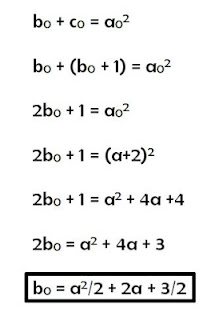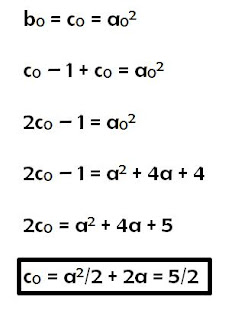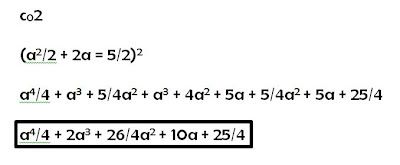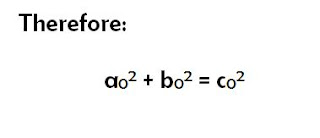Categories

# Pythagorean Triples Part 2: Teacher Learning

If you are not careful, teaching can become very boring, very quickly. Most teachers of specialized areas teach the same content arranged in the same manner numerous times throughout a career. It is no wonder teachers are constantly warned of burnout. Opening up space for student initiative serves a two-fold purpose:

First, the extra freedom allows students to create significance in memorable ways.
Second, the sheer variety of student queries can raise questions for teachers.

Essentially, surprises lead to great student and teacher learning. The Pythagorean triple exercise (detailed in a previous post) is a perfect example.

Pythagorean Triples Part 1: Student Strategies

I left the class with two main questions:

1. Why does multiplying a Pythagorean triple by a constant yield another?
2. Does the method of squaring odd numbers and finding consecutive whole numbers always work?

The first was fairly straightforward. I had seen the number theory proof before; it is quite simple and relies on the use of a greatest common factor. Until this class, I had never stopped to think about how my students would make sense of this fact. I guess I wanted them to think of it as a happy coincidence. I began to ask myself if there were any curricular connections to the idea of non-primitive Pythagorean triple generation.

One student had put it this way:

“The numbers all work because each one is being altered by a constant amount.”

It was almost as though it had been staring me right in the face; I had just found an excellent transition activity between the Pythagorean Theorem and Similar Polygons. Ironically, those two topics appear as consecutive units in my Workplace 10 course this year. By complete chance, I had manufactured an opportunity to combine two seemingly unrelated topics.

When we began similar triangles, I had students construct some of their triples and notice the connections. Until that moment, I had always ignored the geometric implications of the theorem because it is typically presented in an algebraic way throughout high school.

The second question needed to be solved with a piece of paper. I enjoy doing mathematics that relates to my job. Solving unique problems from the fields that I teach gives me a deeper understanding of the discipline. It allows me to approach the classroom from a different light–as someone who holds more than answers. Someone who also holds questions.

I wrote out the proof and digitized it later. Essentially, I define the conditions:From here I used the facts that the new “b” and “c” summed to the square of the new “a” and the new “b” and “c” were consecutive to define both the new “b” and “c” in terms of “a”.I then had all three components of the new triple. I added the squares of the new “a” and “b”and then squared the new “c” to check equalityThe perfect match led to the conclusion that the three new components do indeed create a new triple. What’s more is that because the new “b” and “c” differ by one, they cannot both be multiples of a number larger than one. So the new triple, if generated by a primitive triple, is also primitive.I challenged the students to find an infinite number of Pythagorean triples thinking that they would come up with the fact that multiplying by a constant preserves the relationship. I had no idea that this particular student would find something much deeper.
If teachers aren’t learning anything new themselves, they aren’t looking hard enough.
NatBanting

## 4 replies on “Pythagorean Triples Part 2: Teacher Learning”

Not sure I can do this justice in a comment, but here's a proof that feels easier to me:
Given: a is odd.
Let b=(a^2-1)/2, a is odd guarantees b is a whole #
Let c=(a^2+1)/2, also a whole #
b^2=(a^4-2a^2+1)/4
c^2=(a^4+2a^2+1)/4
c^2-b^2 = 4a^2/4 = a^2 QED

@Sue
That is a far more elegant way of putting it. The reason why I structured the proof in this way is because it mirrored the studnet's thought. He was taking an odd number, squaring and finding two integers. Then testing to see if LHS was equal to RHS. Showing him this structure gelled with his thought.
Things aren't as "easy" when your dealing with a grade 10 student who has never seen number theory.
Thanks for the comment.

If it worked for him, then it's perfect. Would changing mine to the following reflect his process more closely?

Given: a is odd.
Let b=(a^2-1)/2, a is odd guarantees b is a whole #
Let c=(a^2+1)/2, also a whole #
b^2=(a^4-2a^2+1)/4
a^2+b^2 = (a^4-2a^2+1)/4 +4a^2/4 = (a^4+2a^2+1)/4
c^2=(a^4+2a^2+1)/4
So a^2+b^2 = c^2 QED

He might like seeing two different methods.

Here's another way to explain it. Every odd number is the difference of two consecutive squares. In particular, every odd square a^2 is the difference of two consecutive squares c^2 – b^2.